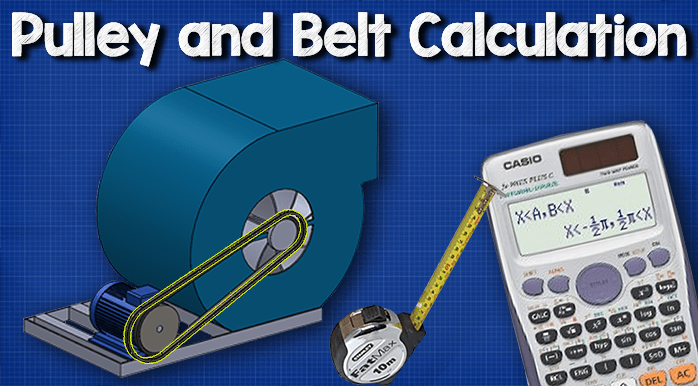# Pulley Belt Calculations

Belt length, distance between pulley wheels

0
2555How to calculate pulley belt length and distance between wheels. In this article we’re going to be looking at some pulley belt calculations with worked examples.

As shown in the image below, an induction motor is connected via a belt to drive two pulleys which will move centrifugal fan. This is this fairly typical of what you might find to in an Air Handling Unit or AHU.

You’d also have this set up on some pumps and also some conveyor belts.

The two things we’ll be calculating in this video are the belt length (how long is, or does that need to be?) And also the distance between the two pulleys, which we’re just going to reverse that calculation to find that.

These calculations are mainly for existing installations but you could also put it towards your design if you want to calculate what the length needs to be of the belt, as long as you already know the distance between the two pulleys and the size of the pulleys. The second part, the distance between the pulleys, is if you already know what length belt you need and also you know the diameter of both the pulleys. But you’re not sure exactly what the distance should be between them.

## Calculate Pulley Belt Length

You can do it manually OR you can download our excel sheet!

I just want to point out that these calculations may vary slightly from the real world scenario, but this is the best calculation that you can perform to get the most accurate figure.

First of all, we’ll have a look at what length dose the pulley belt need to be? In this example, we’re going to look at the belt between the induction motor and also a centrifugal fan in an AHU.

Now, we already know the size of the pulley, either from the manufacturer’s data or we can go out into the building and actually measure this. Remember Pulley 1 is always going to be the largest pulley you have, and you’ll see this as we go through the calculations shortly.

We also have Pulley 2 and we know the diameter of this pulley as well. Again, this is taken from manufacturer’s data or measured. We also know the centre distance between these two pulleys.

The image above shows the formula we’re going to use to calculate this. It looks quite long but it’s not as complex as it might first seem. I’ve also colour coded the numbers, as many as possible, just to make it a little bit easier. And I’ve also split the calculations into metric and imperial. So whichever units of measurement you’re using, you can follow these through.

We can now drop our numbers in. Remember we calculate the brackets first.

## Calculate distance between pulleys

If we need to calculate the distance between the centres of the two pulleys, then we can use the following calculations to approximate this. We will need to know the diameters of the pulleys as well as the belt length.